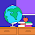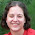## Thursday, May 23, 2013

### MaTh activity Thursday with Percentages

It's Thursday once again and we are doing Percentages!  I know it may be a hard one for you, but I'd love to hear how you use percentages in your class.

I feel that percentages are one of those things that either kids are good at or they are not.  There doesn't seem to be many in the middle.

A child in fourth or fifth grade should be able to understand percentages and they can understand it well, if they are introduced to it in a straightforward manner.

Here is how I teach percentages...

A percentage is just another way we show fractions and decimals, but a percentage is how those fractions and decimals relate to 100.  For example...

• 1/4 is also known as a quarter.
• In decimal form it is 0.25 (That's what a quarter coin in worth--1/4 of a dollar).
• 0.25 equals 25/100 (twenty-five hundredths)
• So, if 25 people out of 100 people like strawberries, we would say 25% of people like strawberries.
Whenever you see a decimal it can be turned into a percentage.  It is just the first two place values below the decimal (the tenths and hundredths spots) . _ _ =  _ _ %

Children will then need practice showing their understanding of this information.

Once they have gotten good at that you can teach them how to find a percentage with any situation.  They do need to have a knowledge of multiplication and division.  They normally teach this in Algebra, but young children can learn it easily...

You know that when you have an equation in order to have the equal sign the equation must be balanced...
2+2=4.  If you had 2+3=4 that wouldn't be right.  It would be 2+3>4 (since you know 2+3 is a total of 5).

In math we put the unknown as an x.
For example: 4+3= x  and you then know that x=7
or 15 ÷ x = 3 and you know that x = 5

Let's try a problem to find the percentage.

7 out of 30 kids in your class like broccoli.  What percentage of the class likes broccoli?

There are a couple ways you can do it.  Simply divide: 7/30 and remember that the decimal you come up needs to be changed to a percentage.

The other way to do it is a bit more complicated.  It is the way you would learn in an algebra class.  You are comparing the situation with the fraction representation of a percentage (something hundredths)...
I know that children at about age 9 and above can understand this, if they have a basic understanding of number concepts of multiplication and division.  In other posts I will show those concepts.1.This is such a great idea! I teach Kindergarten so it's a little out of my area but I look forward to the next one!

My Kinder-Garden

2.Being a history teacher we use percentages to figure out historical data/current data....like how much of the population is _____. Or what is the percentage of presidents from Virginia?

3.Changing percents to decimals and fractions is no longer in my curriculum but a few years ago when I did teach it, I made a powerpoint with characters from the movie Madagascar on the slides as I taught how to change a fraction to percent and percent to fraction. Then when it came time to change decimals to percents and percents to decimals, the song Move It Move It from the movie would come on and when they sang Move it Move It, I clicked my remote and the decimal would bounce across to places. The students loved it and they actually remembered to move the decimal 2 places

Thanks SO much for stopping by! I appreciate your comments and any ideas you share! Have a wonderful day : )